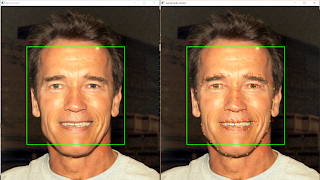# Getting Dlib Face Landmark Detection working with OpenCV

Dlib has excellent Face Detection and Face Landmark Detection algorithms built-in. Its face detection is based on Histogram of Oriented Gradients (HOG) feature combined with a linear classifier, on a sliding window detection scheme (Ref. http://dlib.net/) and it provides pre-trained models for face landmark detection. It also provides handy utility functions like dlib.get_frontal_face_detector() to make our lives easier.Dlib Face Landmark Detection in action
Note: Image used for testing is in the Public Domain - https://en.wikipedia.org/wiki/File:Arnold_Schwarzenegger_edit%28ws%29.jpg

To check out Dlib with it's native functions, you can try out the Dlib example from the official site: http://dlib.net/face_landmark_detection.py.html.It works well, but we can do better.

Although Dlib offers all the simplicity in implementing face landmark detection, it's still no match for the flexibility of OpenCV. (Simply put, Dlib is a library for Machine Learning, while OpenCV is for Computer Vision and Image Processing)

So, can we use Dlib face landmark detection functionality in an OpenCV context? Yes, here's how.

In order for the Dlib Face Landmark Detector to work, we need to pass it the image, and a rough bounding box of the face. The said bounding box doesn't need to be exact, it just helps the landmark detector to orient itself to the face. So, we can use an OpenCV Cascade Classifier with a Haar Cascade to detect a face and use it to get the face bounding box.

Update 12/Apr/2017: The code is now updated so that it works with both OpenCV 2 and 3, and both Python 2.7 and 3+.

First we'll load the required modules, and set the paths for the input image and the models for the detectors,
`````` import numpy as np
import cv2
import dlib

image_path = "path to your image"
predictor_path= "path to your shape_predictor_68_face_landmarks.dat file"
``````

The shape_predictor_68_face_landmarks.dat file is the pre-trained Dlib model for face landmark detection. You can get it from the official Dlib site here: dlib.net/files/shape_predictor_68_face_landmarks.dat.bz2. It's zipped with bz2, so just unzip it to get the .dat file.

Now, we create the OpenCV Cascade classifier, and Dlib shape predictor for landmarks,
`````` # Create the haar cascade

# create the landmark predictor
predictor = dlib.shape_predictor(predictor_path)
``````

We'll then read in the image, and convert it to grayscale,
`````` # Read the image
# convert the image to grayscale
gray = cv2.cvtColor(image, cv2.COLOR_BGR2GRAY)
``````

Then we'll ask OpenCV to look for faces in our image...
`````` # Detect faces in the image
gray,
scaleFactor=1.05,
minNeighbors=5,
minSize=(100, 100),
)
``````

... and enumerate through the found faces,
`````` print("Found {0} faces!".format(len(faces)))

# Draw a rectangle around the faces
for (x, y, w, h) in faces:
cv2.rectangle(image, (x, y), (x + w, y + h), (0, 255, 0), 2)
``````

Now, we need to get the bounding box for each face in Dlib Rectangle format. So we create a Dlib Rectangle object from the OpenCV coordinates,
`````` # Converting the OpenCV rectangle coordinates to Dlib rectangle
dlib_rect = dlib.rectangle(int(x), int(y), int(x + w), int(y + h))
``````

We use that rectangle as the bounding box to detect the face landmarks, and extract out the coordinates of the landmarks so OpenCV can use them,
`````` detected_landmarks = predictor(image, dlib_rect).parts()

landmarks = np.matrix([[p.x, p.y] for p in detected_landmarks])
``````

We then enumerate through the landmark coordinates and mark them on the image,
`````` # copying the image so we can see side-by-side
image_copy = image.copy()

for idx, point in enumerate(landmarks):
pos = (point[0, 0], point[0, 1])

# annotate the positions
cv2.putText(image_copy, str(idx), pos,
fontFace=cv2.FONT_HERSHEY_SIMPLEX,
fontScale=0.4,
color=(0, 0, 255))

# draw points on the landmark positions
cv2.circle(image_copy, pos, 3, color=(0, 255, 255))
``````

Finally, we draw the annotated image on an OpenCV window,
`````` cv2.imshow("Faces found", image)
cv2.imshow("Landmarks found", image_copy)
cv2.waitKey(0)
``````

The result is satisfying,Dlib Face Landmarks on OpenCV
Here's the full code for your convenience,
`````` import numpy as np
import cv2
import dlib

image_path = "path to your image"
predictor_path= "path to your shape_predictor_68_face_landmarks.dat file"

# create the landmark predictor
predictor = dlib.shape_predictor(predictor_path)

# convert the image to grayscale
gray = cv2.cvtColor(image, cv2.COLOR_BGR2GRAY)

# Detect faces in the image
gray,
scaleFactor=1.05,
minNeighbors=5,
minSize=(100, 100),
)

print("Found {0} faces!".format(len(faces)))

# Draw a rectangle around the faces
for (x, y, w, h) in faces:
cv2.rectangle(image, (x, y), (x + w, y + h), (0, 255, 0), 2)

# Converting the OpenCV rectangle coordinates to Dlib rectangle
dlib_rect = dlib.rectangle(int(x), int(y), int(x + w), int(y + h))
print dlib_rect

detected_landmarks = predictor(image, dlib_rect).parts()

landmarks = np.matrix([[p.x, p.y] for p in detected_landmarks])

# copying the image so we can see side-by-side
image_copy = image.copy()

for idx, point in enumerate(landmarks):
pos = (point[0, 0], point[0, 1])

# annotate the positions
cv2.putText(image_copy, str(idx), pos,
fontFace=cv2.FONT_HERSHEY_SIMPLEX,
fontScale=0.4,
color=(0, 0, 255))

# draw points on the landmark positions
cv2.circle(image_copy, pos, 3, color=(0, 255, 255))

cv2.imshow("Faces found", image)
cv2.imshow("Landmarks found", image_copy)
cv2.waitKey(0)

``````

Update 12/Apr/2017: Dlib is quite flexible when used with OpenCV. You can even access each of the facial features individually from the 68 landmarks. Check out my new post on How to access each facial feature individually from Dlib.

https://matthewearl.github.io/2015/07/28/switching-eds-with-python/

Build Deeper: Deep Learning Beginners' Guide is the ultimate guide for anyone taking their first step into Deep Learning.

1.hi i am facing this error please do help me ! :(
Traceback (most recent call last):
File "/home/pi/fypcoding2/real-time-facial-landmarks/real-time-facial-landmarks/videofacelandmarks.py", line 13, in
predictor = dlib.shape_predictor(predictor_path)
RuntimeError: Error deserializing a floating point number.
while deserializing a dlib::matrix
while deserializing object of type std::vector
while deserializing object of type std::vector
while deserializing object of type std::vector

2.hi,
im getting this error
RuntimeError Traceback (most recent call last)
in ()
3
4 # create the landmark predictor
----> 5 predictor = dlib.shape_predictor(predictor_path)

RuntimeError: Unable to open E/java edx/shape_predictor_68_face_landmarks.dat
please have a look over it

3.4.5.Thank you so much...